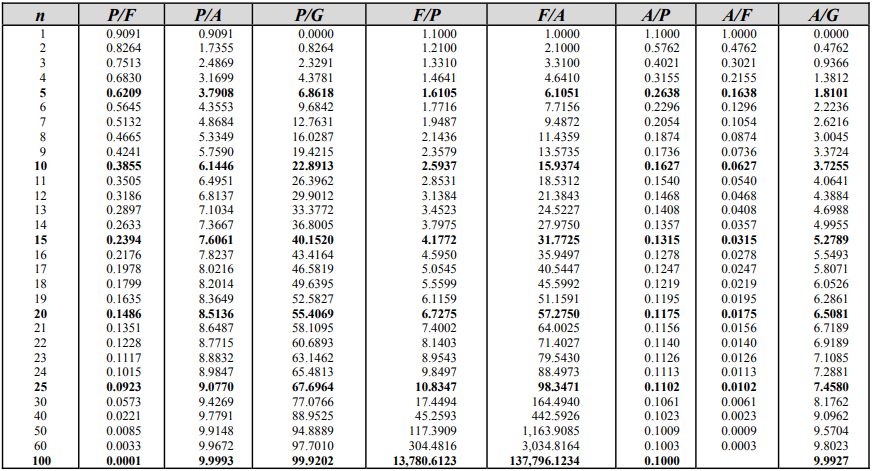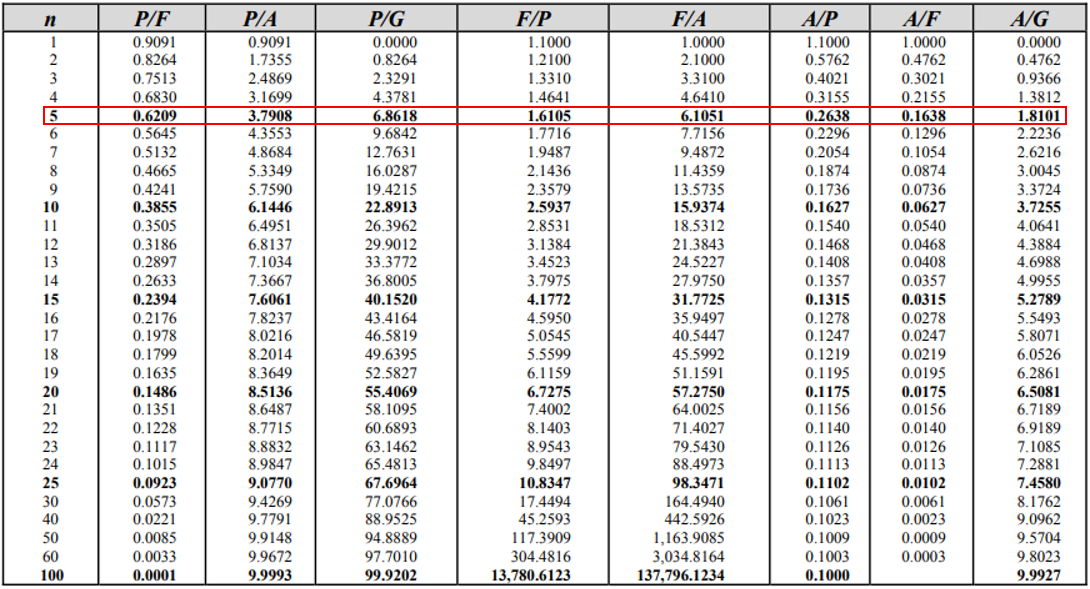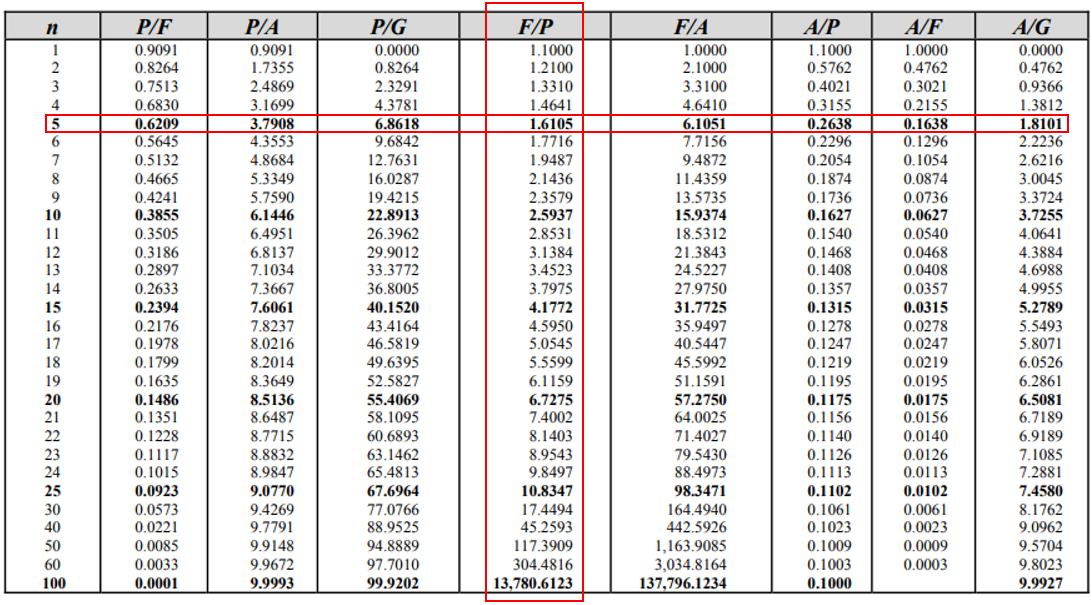## Interest Rate Table

Using the interest rate table below, how much money would have accrued after 5 years if $2,000 is invested now at 10% compounded annually?Hint The problem statement is asking for growth after 5 years, so $$n=5$$ :Hint 2 We are trying to solve for the Future, $$F$$ , given the present value, $$P$$ , of$2,000.The problem statement is asking for a single payment based on a compounded amount. Based on the givens: $$n$$ , the number of compounding periods, is 5. We are trying to solve for the Future, $$F$$ , given the present value, $$P$$ , which converts to $$F\:given\:P$$ .The intersection value, 1.6105, from the 10% Interest Rate Table is the compounding factor. Thus, the initial investment will grow into:
$$Future=Present \times Factor$$$$$Future=\2,000 \times 1.6105=\3,221$$$
Note, we can check our answer with the below formula:
$$(1+i)^n=(1+0.1)^5=(1.1)^5=1.6105$$$where $$i$$ is the interest rate, and $$n$$ is the number of compounding periods. Because our compounding factor matches with the table, we confirmed that our initial answer is correct.$3,221